# Quantization and Noise

October 23, 2019

### Uniform 8-bit Quantization

A typical analog to digital converter (ADC) performs quantization 𝖰[𝗒(𝑛)] on a sample 𝗒(𝑛) as illustrated in Figure 4.1. From there, the input peak-to-peak range 𝑅 is partitioned into 23 = 8 intervals all of equal length 𝛥.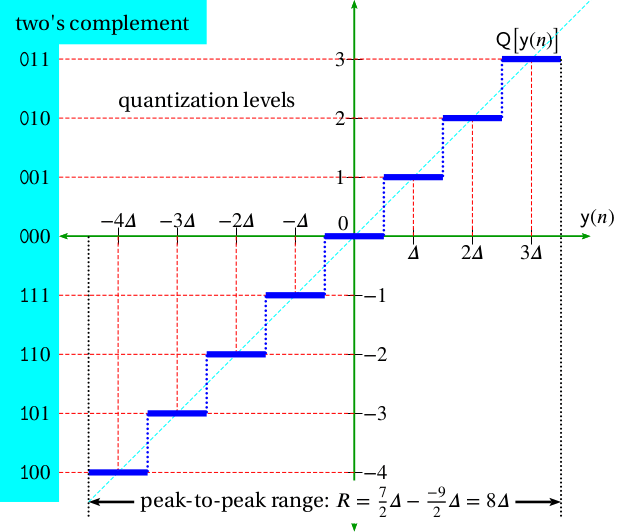Figure 4.1. Quantization 𝖰[𝗒(𝑛)] on a sample 𝗒(𝑛).

Quantizers may be either midtread or midrise and either uniform or non-uniform. In practice, midtread tends to be more popular because, unlike midrise, a small signal 𝗒(𝑛) about 0 causes no jitter in the quantization sequence 𝖰[𝗒(𝑛)]. In this document, we focus on uniform midtread quantization.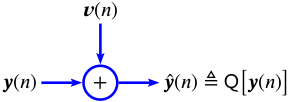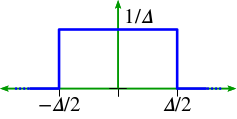### Quantization Noise Model

Quantization results in a distortion that is referred to as quantization noise. Quantization noise can be modeled, more or less accurately, as uniformly distributed additive white noise as described below.

Quantization is defined as additive noise 𝒗(𝑛) and with these assumptions:

• 𝒗(𝑛) and 𝒗(𝑚) are uncorrelated for 𝑚 ≠ 𝑛
• Each 𝒗(𝑛) is uniformly distributed on the interval [𝛥/2 ∶ 𝛥/2).
• 𝒚(𝑛) ≜ 𝒙(𝑛𝜏) and 𝒗(𝑛) are uncorrelated.

Let 𝒗(𝑛) be quantization noise as defined above. Then, 𝖵𝖺𝗋𝒗(𝑛) = 𝛥2/12.

#### Proof

(1)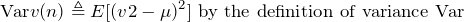(2)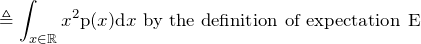(3)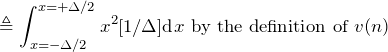(4)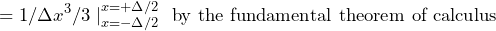(5)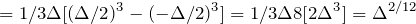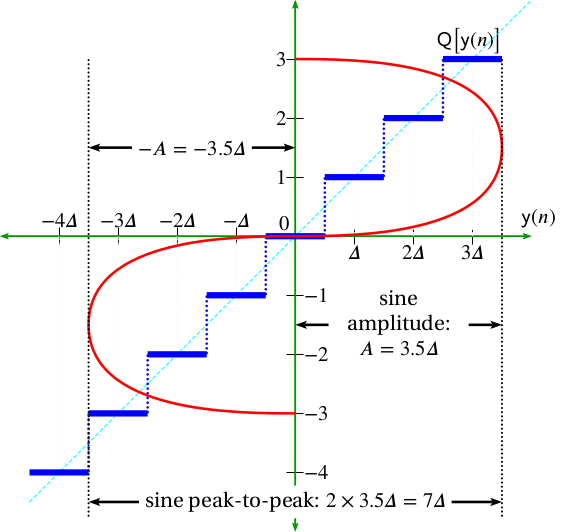Full-scale sine quantized using a uniform midtread quantizer.